#### You may also like### Tri.'s

How many triangles can you make on the 3 by 3 pegboard?### Cutting Corners

Can you make the most extraordinary, the most amazing, the most unusual patterns/designs from these triangles which are made in a special way?### Bracelets

Investigate the different shaped bracelets you could make from 18 different spherical beads. How do they compare if you use 24 beads?

# Name That Triangle!

##### Age 7 to 11Challenge Level

Anabelle from Waverley Christian College in Australia sent in the following: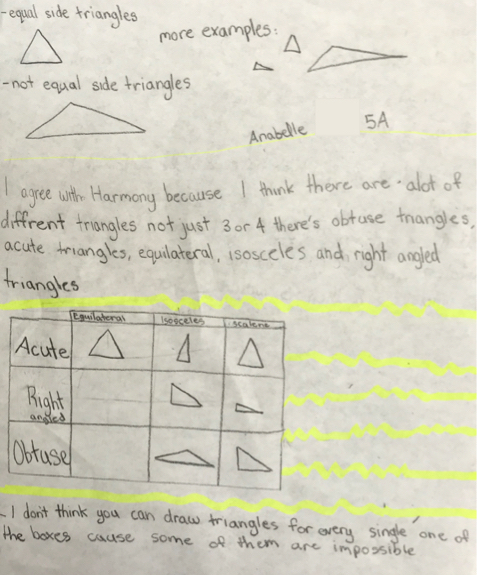Felicity, Siya and Zara from North London Collegiate Junior School sent in this explanation:

Enclosed is our solution for Name that Triangle! that we did together in our after-school maths club.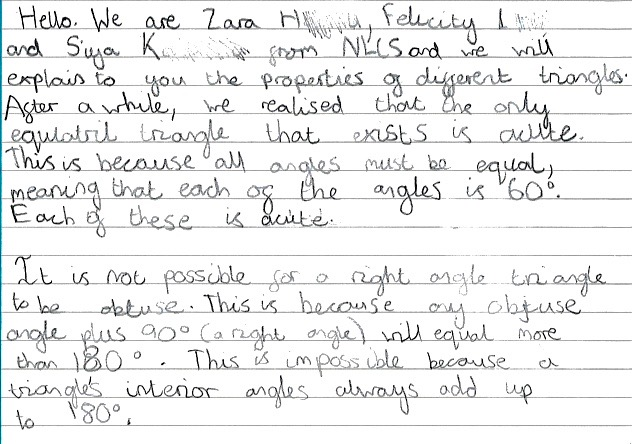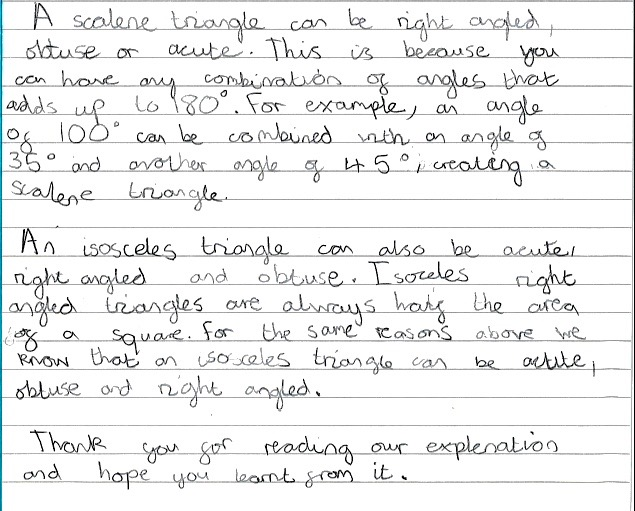Sofia, Jasmin, Misha, Trina, Mia and Melania, and Mohinika with Tejaswini, also from London Collegiate Junior School, sent in the following: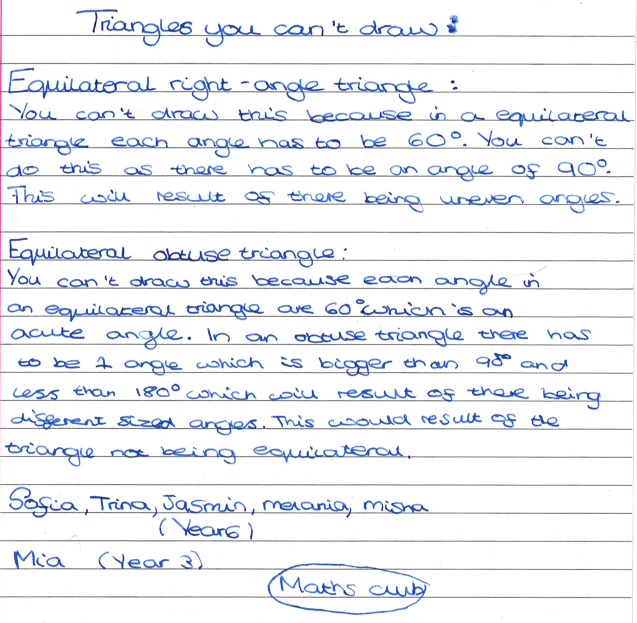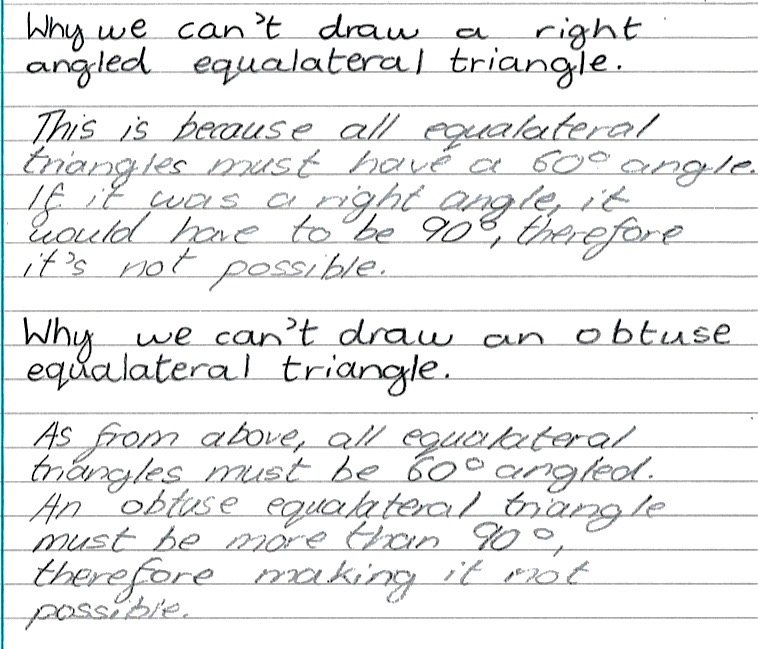Scout from James Allen's Prep School began by explaining that the angles of any triangle must add up to 180 degrees and wrote explained carefully why each type of triangle is or is not possible:

Acute + Equilateral = Definite (The angles of an equilateral triangle are all 60 degrees which makes them all acute, meaning they are all smaller than 90 degrees.)

Acute + Isosceles = Possible (An isosceles triangle could be two acute or all acute. Here are some examples: 30 degrees, 30 degrees and 120 degrees or 75 degrees, 75 degrees and 30 degrees. One acute angle is not possible because you would have to have two obtuse or right angles and that alone would be more than the limit.)

Acute + Scalene = Possible (Because a scalene triangle's angles must be all different and here are some examples: 59 degrees, 60 degrees and 61 degrees or 50 degrees, 60 degrees and 70 degrees.)

Right angled + Isosceles = Possible (If you have 90 degrees, 45 degrees and 45 degrees that would work, but no other combination would. The two equal angles must be the same and in this case, we need a right angle; the equal angles cannot be 90 degrees, as 90 + 90= 180, which is the limit of triangles.)

Right angled + Equilateral = Impossible (The underlying principle of an equilateral triangle is that its angles are the same. To get them to be the same they all must be 60 degrees and a right angle is 90 degrees.)

Right angled + Scalene = Possible (If you have 90 degrees, 30 degrees and 60 degrees that is Scalene and is just one of many. In fact, with a right angle, you can just keep one angle 90 degrees and the other two can be anything if together they add up to 90 degrees and aren't both 45 degrees.)

Obtuse + Equilateral = Impossible (In order for all angles to be the same, each angle must be 60 degrees. 60 degrees is less than 90 degrees, so not obtuse.)

Obtuse + Isosceles = only possible with one obtuse angle (It depends whether you see obtuse as three obtuse or two obtuse (both impossible as it is over the limit: 180 degrees). One is possible: 120 degrees, 30 degrees and 30 degrees is just one example.)

Obtuse + Scalene = only one obtuse angle possible (A scalene can have only one obtuse angle to stay within the limit. For example, 120 degrees, 40 degrees and 20 degrees.)

Thank you, Scout, a very thorough explanation, well done!